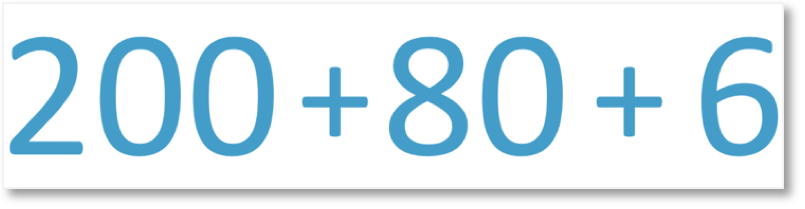# Hundreds, Tens and Units

Hundreds, Tens and Units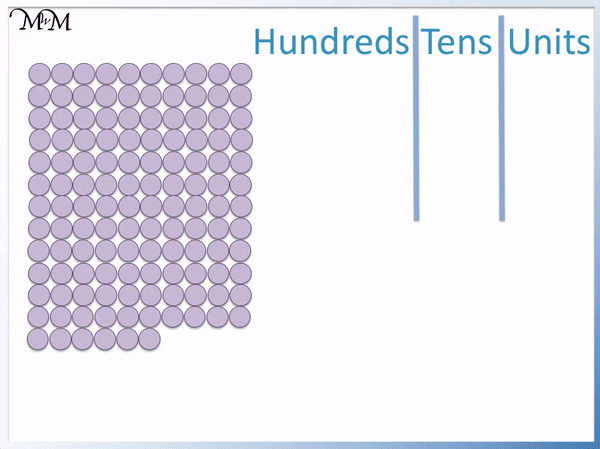• We can count these counters more easily by collecting them into groups of hundreds, groups of ten and groups of units.
• ‘Unit’ means ‘one’ and each counter is one unit.
• Each contains a group of ten units.
• We can count ten rows of ten units to make a total square of one hundred counters.
• We only have one group of one hundred counters and therefore we write a ‘1’ in the hundreds column of our place value chart.
• There are two rows of ten remaining so we can write a ‘2’ in the tens column of our place value chart.
• We have six counters remaining so we write a ‘6’ in the units (or ones) column.
• The total number is 126.
• We form our number from the .
It is easier to count large numbers by counting the groups of hundreds, groups of tens and groups of ones.• We can count the total number of counters here by counting the groups of hundreds, tens and units.
• We have 4 groups of hundreds.
• We have 6 groups of ten.
• We finally have 3 units (or ones).
• We write our total by combining these : 4, 6 and 3 in order.
• The total number of counters is 463.Supporting Lessons# What are Hundreds, Tens and Units (Ones)?

Hundreds, tens and units are used to help us count large numbers more easily. We write a to represent how many hundreds, tens and units we have. We form our total number by combining these digits in order.

Almost all of the numbers that we use in life are represented in this manner, by counting groups of ten and groups of one hundred and then writing down how many we have.

To visualise the numbers in this lesson we will use counters.

Each counter will be worth one.

Therefore the total number that we have will be the total number of ‘ones’ that we have.

The word ‘unit’ means ‘one’ and is also used for counting groups of numbers. So we can count the ‘units’ that we have. Each counter is one unit.

When teaching place value, some schools and teachers will prefer to say ‘ones’, whilst others will prefer to say ‘units’. Both terms are correct.

Counting all of these counters one by one would be quite slow and in these lessons we will be looking at how to count large groups of numbers by collecting them into groups.

Specifically we will count how many hundreds we have and how many tens we have.

Below, we have a group of counters, arranged in a square.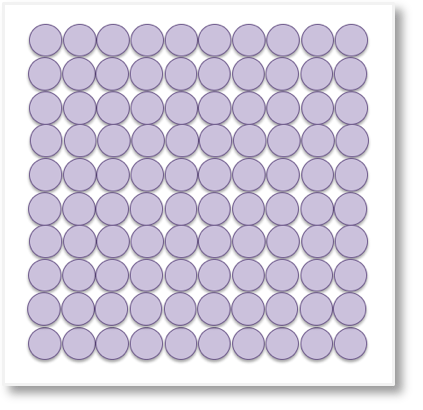In order to begin counting the number of counters, we put them into groups of ten and then count how many of these groups of ten that we have.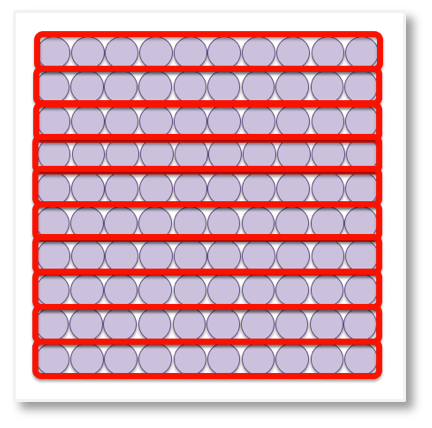Here, we have 10 groups of ten in total.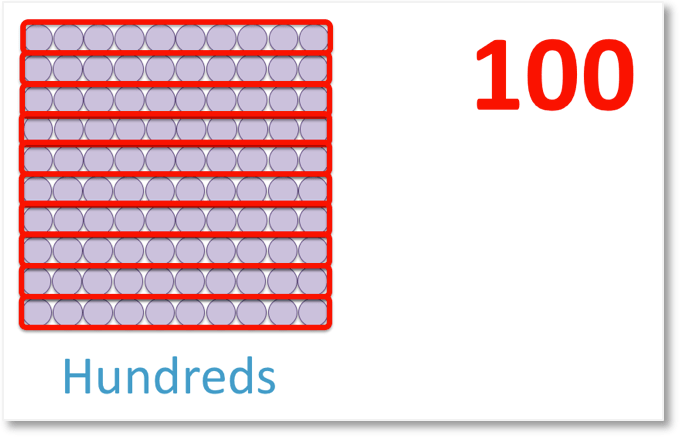This gives us a total of 100.

Instead of counting these as 10 groups of ten, we can say that we have 1 hundred. By collecting 100 units into a square helps us identify groups of one hundred. We simply need to gather the counters into a 100 square and then count how many squares we have. Each square is worth 100.

We will count how many hundreds we have, then count how many groups of ten are left and finally see how many remaining units there are.

Here is an example where we will write the totals in place value columns:To write this total number using

, we can begin by grouping the counters into tens.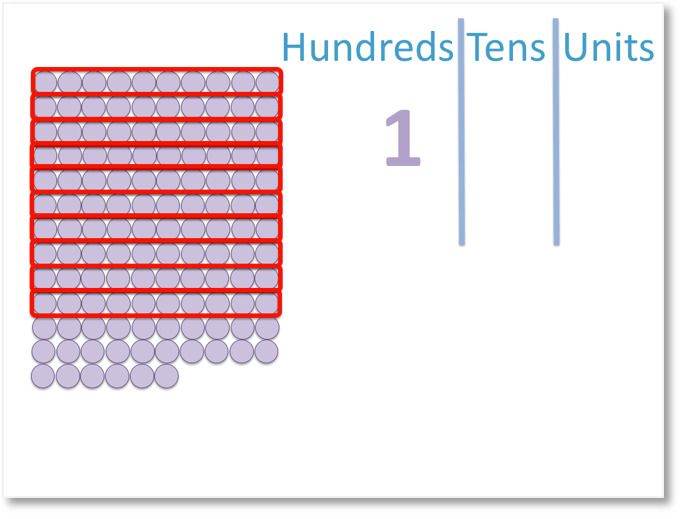Here, we have found and circled 10 groups of ten, which is the same as 1 group of one hundred.

So, we write a 1 in the hundreds column.

We can continue to group any tens that we have.We have 2 more groups of ten. So, we can write a 2 in the tens column.There are 6 individual unit counters left over. So, we write a 6 in the units column.

We have the number 126 (one hundred and twenty-six).

Here is another example of using hundreds, tens and units to help us quickly count a large number.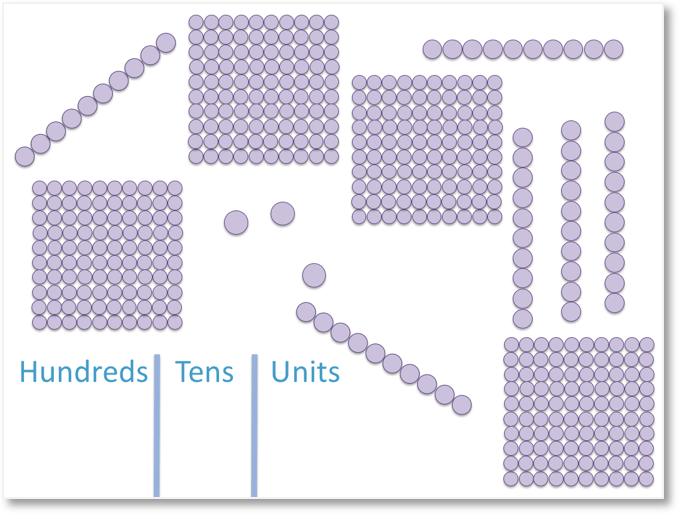We can represent the counters above in numerals, by counting the number of groups of hundreds, tens and units.

We begin by counting how many hundreds we have. We have collected the groups of hundred counters into squares, so that they are easier to count.

There are 4 hundreds, so we write a 4 in the hundreds column.Next, we count how many groups of ten units we have.There are 6 tens, so we write a 6 in the tens column.

Finally, we count how many units we have.There are 3 units remaining, so we write a 3 in the units column.

The digits in the place value columns then form our total number.

We have 4, 6 and then 3.

Therefore, we have the number 463 (four hundred and sixty-three).

The digit ‘4’ is in the hundreds place value column and therefore represents 400 (four hundred), not just four.

The digit ‘6’ is in the tens column and is therefore worth 6 tens which is 60.

The rightmost digit is ‘3’ and since it is in the units column it is just worth 3.

When teaching hundreds, tens and units it is important to introduce this topic using physical objects such as counters or base ten blocks.

We have hundreds, tens and units worksheets above and we also have provided blank hundreds, tens and units (or ones) place value charts above that you can print off and use to represent numbers.

You can move the counters or base ten blocks into the columns of these worksheets.Now try our lesson on Partitioning Hundreds, Tens and Units where we learn how to split our number into its hundreds, tens and units.